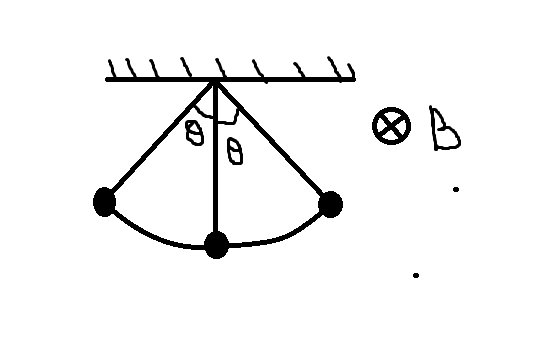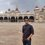Magnetics!!!A simple Pendulum with a charged bob is oscillating as shown in figure. Time period of oscillation is $T$ and the angular amplitude is $\theta$. If a uniform magnetic field perpendicular to the plane of oscillation is switched on, then

$(a)$ $T$ will decrease but $\theta$ will remain constant.

$(b)$ $T$ will remain constant but $\theta$ will decrease.

$(c)$ Both $T$ and $\theta$ will remain the same.

$(d)$ Both $T$ and $\theta$ will decrease.Note by Advitiya Brijesh
6 years, 4 months ago

This discussion board is a place to discuss our Daily Challenges and the math and science related to those challenges. Explanations are more than just a solution — they should explain the steps and thinking strategies that you used to obtain the solution. Comments should further the discussion of math and science.

When posting on Brilliant:

• Use the emojis to react to an explanation, whether you're congratulating a job well done , or just really confused .
• Ask specific questions about the challenge or the steps in somebody's explanation. Well-posed questions can add a lot to the discussion, but posting "I don't understand!" doesn't help anyone.
• Try to contribute something new to the discussion, whether it is an extension, generalization or other idea related to the challenge.
• Stay on topic — we're all here to learn more about math and science, not to hear about your favorite get-rich-quick scheme or current world events.

MarkdownAppears as
*italics* or _italics_ italics
**bold** or __bold__ bold

- bulleted
- list

• bulleted
• list

1. numbered
2. list

1. numbered
2. list
Note: you must add a full line of space before and after lists for them to show up correctly
paragraph 1

paragraph 2

paragraph 1

paragraph 2

> This is a quote
This is a quote
# I indented these lines
# 4 spaces, and now they show
# up as a code block.

print "hello world"
# I indented these lines
# 4 spaces, and now they show
# up as a code block.

print "hello world"
MathAppears as
Remember to wrap math in $$...$$ or $...$ to ensure proper formatting.
2 \times 3 $2 \times 3$
2^{34} $2^{34}$
a_{i-1} $a_{i-1}$
\frac{2}{3} $\frac{2}{3}$
\sqrt{2} $\sqrt{2}$
\sum_{i=1}^3 $\sum_{i=1}^3$
\sin \theta $\sin \theta$
\boxed{123} $\boxed{123}$

Sort by:

unrelated. but I love your microsoft paint skills

- 6 years, 4 months ago

why unrelated??....but i too appreciate his skill in "painting"....:)....but a suggestion to him::: he could have used "text" option to write the letter "B" in ms. paint.....:-)

- 6 years, 4 months ago

hmm... next tym i will!!! :P

- 6 years, 4 months ago

:P LOL!!!

- 6 years, 4 months ago

hahaha but I don't think that helped him much!! I think the answer's (e)

- 6 years, 4 months ago

LOL!!

- 6 years, 4 months ago

If you consider in terms of 'energy consevation', work done by 'magnetic field' is $0$. Hence, $\theta$ remains same and so or more time also.

- 6 years, 4 months ago

Since, the charged particle is undergoing a periodic oscillation it will be produce only electric field as the displacement is zero after 1 time period!!! Thus, electric field will be opposed by magnetic field by Lenz's law and Lorentz force concept: lets see how.... F=q.E+(-q.vxB) Now see the net force acting on the charged particle is getting reduced by damping force from the magnetic field!!! So, it will oscillate only to balance tension of the string with perpendicular gravitational force acting on it!!! My idea that time period T of oscillation will decrease but \theta\ will remain constant!!! And moreover vXB is maximum on account that B is perpendicular to the plane of oscillation!! So, it is option a)!!!

- 6 years, 4 months ago

Oscillating charge produces electric field which in turn produces oscillating magnetic field which shoud be true according to you, sir. But one thing to note is that the charges are in 'constantly accelerated' motion, which in this case is equal to $0$.

- 6 years, 4 months ago

I guess (c) is correct. Is it right?

- 6 years, 4 months ago

yes bt how?

- 6 years, 4 months ago

Since the restoring force which is mg $\sin$$\theta$ ('$\theta$' as depicted in your diagram) remains the same so will $\omega$ i.e. angular frequency and so will T. Using energy conservation $\theta$ will also remain same since work done by magnetic force is zero.

- 6 years, 4 months ago

$(c)$ is the correct option... I need explanation!!

- 6 years, 4 months ago